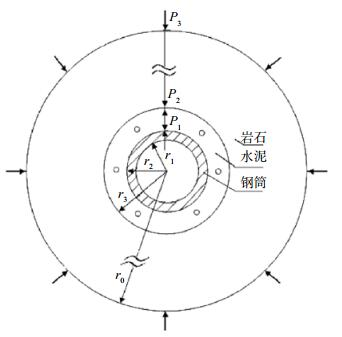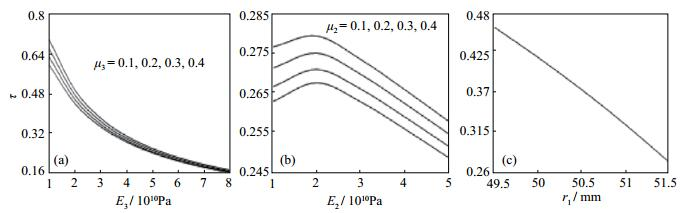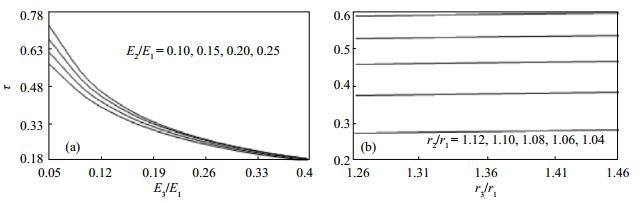﻿ 岩石压应力传递系数计算及影响机制文章快速检索
 地震地磁观测与研究2018, Vol. 39Issue (1): 102-106  DOI: 10.3969/j.issn.1003-3246.2018.01.0170

### 引用本文Zhang Lingkong, Niu Anfu, Huang Fuqiong. Calculation of rock compressive pressure transfer coefficient and its influence mechanism[J]. Seismological and Geomagnetic Observation and Research, 2018, 39(1): 102-106. DOI: 10.3969/j.issn.1003-3246.2018.01.017.

### 文章历史

Calculation of rock compressive pressure transfer coefficient and its influence mechanism
Zhang Lingkong, Niu Anfu, Huang Fuqiong
China Earthquake Networks Center, Beijing Municipality 100045, China
Abstract: Borehole strain observation is the main method to measure the strain of the crust and rock. Study on the additional stress field can effectively analyze the rock compressive stress to transfer probe. The study of the design and mechanical properties of the corresponding parameters of the instrument and the physical interpretation of the observed data can provide help. Considering the applied stress transfer in the rock, cement and equipment steel tube and other 3 layers of medium, according to the thick wall cylinder equation in elastic mechanics, the calculation formula of transmission coefficient of rock stress under the action of axial symmetrical stress is derived. The influence mechanism is analyzed. And the relevant change rule curve and quantitative index are given.
Key Words: borehole strain measurement    rock compressive stress    transfer coefficient    thick walled cylinder equation
0 引言

1 岩石压应力传递系数计算图 1 钻孔应变观测3层介质力学模型 Fig.1 Three layer medium mechanics model of borehole strain observation
 $k = \frac{{{\varepsilon _{\rm{m}}}}}{{{\varepsilon _{{\rm{m0}}}}}} = \frac{{r_2^2{E_3}{P_1}}}{{\left({r_2^2 - r_1^2} \right){E_1}{P_3}}}$ (1)

 $k = {x_4}{E_3}/{E_1}$ (2)

 ${x_4} = \frac{{r_2^2r_3^2\left({{\alpha _2} + {\beta _2}} \right)\left({{\alpha _3} + {\beta _3}} \right)}}{{r_2^2\left({{\alpha _2} - {\alpha _3}} \right)\left[ {r_1^2\left({{\alpha _1} + {\beta _2}} \right) + r_2^2\left({{\beta _1} - {\beta _2}} \right)} \right] + r_3^2\left({{\alpha _3} + {\beta _2}} \right)\left[ {r_1^2\left({{\alpha _1} - {\alpha _2}} \right) + r_2^2\left({{\alpha _2} + {\beta _1}} \right)} \right]}}$ (3)

 $\tau = {P_1}/{P_3} = \left({r_2^2 - r_1^2} \right){E_1}k/\left({r_2^2{E_3}} \right) = \left[ {1 - {{\left({{r_1}/{r_2}} \right)}^2}} \right]k\;{E_1}/{E_3}$ (4)

 $\tau = \left[ {1 - {{\left({{r_1}/{r_2}} \right)}^2}} \right]{x_4}$ (5)

 $\tau = {P_1}/{P_3} = \left({{P_2}/{P_3}} \right)\left({{P_1}/{P_2}} \right) = {\tau _{\rm{s}}}{\tau _0}$ (6)

r21 = r2/r1, r31 = r3/r1E21 = E2/E1E31 = E3/E1，则由式（5）可得

 $\tau = \left[ {1 - {{\left({1/{r_{21}}} \right)}^2}} \right]{x_4}$ (7)

 ${x_4} = 4r_{21}^2\;r_{31}^2/\left({{E_{21}}\;{E_{31}}Z} \right)$ (8)

 $\begin{array}{l} Z = r_{21}^2\left({\frac{{1 + {\mu _2}}}{{{E_{21}}}} - \frac{{1 + {\mu _3}}}{{{E_{31}}}}} \right)\left[ {1 + {\mu _1} + \frac{{1 - {\mu _2}}}{{{E_{21}}}} + r_{21}^2\left({1 - {\mu _1} - \frac{{1 - {\mu _2}}}{{{E_{21}}}}} \right)} \right] + \\ \;\;\;r_{31}^2\left({\frac{{1 - {\mu _2}}}{{{E_{21}}}} + \frac{{1 + {\mu _3}}}{{{E_{31}}}}} \right)\left[ {1 + {\mu _1} - \frac{{1 - {\mu _2}}}{{{E_{21}}}} + r_{21}^2\left({1 - {\mu _1} + \frac{{1 + {\mu _2}}}{{{E_{21}}}}} \right)} \right] \end{array}$ (9)

2 压应力传递系数（τ）影响机制 2.1 τ与岩石弹性模量、泊松系数及钢筒内半径的关系图 2 τ与岩石、水泥的弹性模量（E3、E2）和泊松系数（μ3、μ2）及钢筒内半径（r1）的关系 Fig.2 The relationship between τ and elastic modulus of rock, cement (E3, E2) and Poisson's coefficient (μ3, μ2) and steel cylinder radius (r1)
2.2 τ与水泥的弹性模量和泊松系数的关系

2.3 τ与钢筒内半径或壁厚的关系

2.4 τE3/E1E2/E1的关系

r21= r2/r1= 53.5/ 51.5 = 1.039，r31= r3/r1 = 65/51.5 = 1.262，根据式（7）可以绘制τ值与E3/E1E2/E1的关系曲线，见图 3(a)，图中4条曲线自上而下分别为E2/E1 = 0.10、0.15、0.20、0.25，可知τE3/E1E2/E1的增加而减小，但前者影响显著，后者影响较小，且当E3/E1＞0.26时，E2/E1数值的大小对τ值的影响已无明显差别。图 3 τ与E3/E1、E2/E1及r3/r1、r2/r1的关系 Fig.3 The relationship between τ and E3/E1, E2/E1 and r3/r1 and r2/r1
2.5 τ值与r3/r1r2/r1的关系

E21 = E2/E1 = 3/19.6 = 0.153 1，E31 = E3/E1 = 5/19.6 = 0.255 1，根据式（7）绘制τr3/r1r2/r1的关系曲线，见图 3(b)，图中5条曲线自上而下r2/r1分别为1.12、1.10、1.08、1.06、1.04，可知τr3/r1的增加而缓慢增大，随r2/r1的增加而显著增大。

3 结论

（1）钻孔外围岩石（P3）通过水泥层向应变仪钢筒外壁（P1）的压应力传递系数可表述为τ = P1/P3 = [1-(r1/r2)2] k E1/E3，故P1 = τ P3，其中k为钢筒内壁面应变与空孔岩石面应变之比，是与观测系统（岩石、水泥、钢筒）的各项参数均有关的常数。通常，τ ≈ 0.182 0—0.719 5＜1，故P1P3，即岩石压应力在传递过程中会有所衰减，τ实际反映了P3的传递效率。

（2）τ随岩石弹性模量（E3）和泊松系数（μ3）的增大而下降；水泥弹性参数（E2μ2）的改变对τ影响较小；钢筒内半径（r1）增大或壁厚变薄时，τ值显著下降；τE3/E1E2/E1的增加而减小，随r3/r1r2/r1的增加而增大。

（3）由于基岩并非均匀完整，探头与孔壁耦合也不完善，在实际测量中，岩石压应力沿钻孔可能不是均匀分布，理论计算结果可能存在误差，但因钻孔孔径较小（通常r3 ≤ 76 mm），估计误差不会太大，有待今后进一步验证。

（4）井下岩石弹性参数的测定是一项重要的基础工作，钻孔应变观测的有关理论计算经常要用到它，但目前绝大多数台站未开展该项工作。为了充分利用钻孔应变观测资料，建议安装应变仪探头时，相关单位能给予足够的资金支持，以保障科研任务的顺利开展。

 李海亮, 李宏. 钻孔应变观测现状与展望[J]. 地质学报, 2010, 84(6): 895-900. 刘序俨, 王紫燕, 方宏芳. 对当前四分量钻孔应变观测的审视——以应变不变量为标尺[J]. 地球物理学报, 2014, 57(10): 3332-3346. DOI:10.6038/cjg20141020 牛安福, 张凌空, 闫伟, 等. 中国钻孔应变观测能力及在地震预报中的应用[J]. 大地测量与地球动力学, 2011, 31(2): 48-52. 欧阳祖熙, 张宗润. 钻孔应变仪与井壁耦合方法的研究[M]//赵国光. 地壳构造与地壳应力文集(2). 北京: 地震出版社, 1988. 欧阳祖熙, 张钧, 陈征, 等. 地壳形变深井综合观测技术的新进展[J]. 国际地震动态, 2009, 11061106(1): 1-13. 邱泽华, 石耀霖. 国外钻孔应变观测的发展现状[J]. 地震学报, 2004, 26(Z1): 162-168. 邱泽华. 中国分量钻孔地应力-应变观测发展重要事件回顾[J]. 大地测量与地球动力学, 2010, 30(5): 42-47. 苏恺之, 刘瑞民, 裴玉珍, 等. 体积式钻孔应变仪与岩孔的耦合技术[M]//国家地震局地壳应力研究所. 地壳构造与地壳应力文集(1). 北京: 地震出版社, 1987. 苏恺之, 李海亮, 张均, 等. 钻孔地应变观测新进展[M]. 北京: 地震出版社, 2003. 张宝红. 美国的板块边界观测(PBO)计划[J]. 大地测量与地球动力学, 2004, 24(3): 105-108. 张宝红. 美国板块边界观测(PBO)中的钻孔应变观测设备[J]. 大地测量与地球动力学, 2010, 30(Z2): 40-44. 张凌空, 牛安福. 钻孔体应变与面应变观测参数k的计算[J]. 中国地震, 2013, 29(3): 335-346. Roeloffs E. Tidal calibration of Plate Boundary Observatory borehole strainmeters:Roles of vertical and shear coupling[J]. J Geophys Res, 2010, 115(B6): B06405 Sacks I S, Suyehiro S, Evertson D W. Sacks-Evertson strainmeter, its installation in Japan and some preliminary results concerning strain steps[J]. Proceedings of the Japan Academy, 1971, 47(9): 707-712.